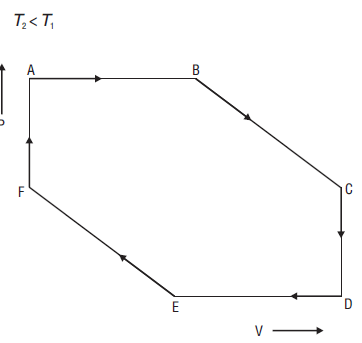##The branch of science which deals with quantitative relation-sheep between heat & other forms of energies is  thermodynamics. To be specific,
It explains how thermal energy is change to  other forms of energy and how matter is affect by this process.
And Thermal energy is the energy that comes from heat and heat is comeout by the movement of tiny particles within an object. like faster these particles move, the more heat is generate

## Thermodynamics Timeline:• Classical Thermodynamics –We analyze the behaviour of matter with a macroscopic approach. We take units such as temperature and pressure into consideration which helps the individuals to calculate other properties and to predict the characteristics of the matter that is undergoing the process.
• Statistical Thermodynamics –Every molecule is under the spotlight i.e. the properties of each and every molecule and ways in which they interact are taken into consideration to characterize the behaviour of a group of molecules.
•  Pure Component Thermodynamics – It tries to describe the behaviour of a system that has an unadulterated or pure constituent.
• Solution Thermodynamics – This section attempts to describe the behaviour of a system that contains more than one chemical in the mixture.

### Some Important Terms Related to Thermodynamics

• System:  It refers to the part of the universe in which observations are carried out.
• Surroundings:  The part of the universe other than the system is known as the surroundings.
• Boundary:  The wall that separates the system from the surroundings is called boundary• Thermodynamic equilibrium: A system in which the macroscopic properties do not undergo any change with time is called thermodynamic equilibrium.
•  Thermal equilibrium: If there is no flow of heat from one portion of the system to another, the system is said to be in thermal equilibrium.
• Mechanical equilibrium: If no mechanical work is done by one part of the system on another part of the system. It is said to be in mechanical equilibrium. Such a condition exists when pressure remains constant.

#### Types of Systems

•  Open system: The system in which energy and matter both can be exchanged with the surroundings.
•  Closed system: The system in which only energy can be exchanged with the surroundings.
•  Isolated system: The system in which neither energy nor matter can be exchanged with the surroundings.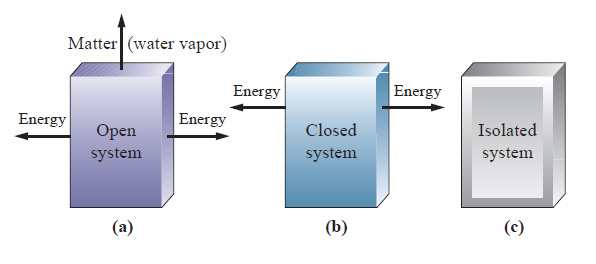#### Thermodynamics Properties:

1. Intensive Properties: Properties of the system which depend only on the nature of matter but not on the quantity of matter are called Intensive properties, e.g., pressure, temperature, specific heat, etc
2. Extensive Properties: Properties of the system which are dependent on the quantity of matter are called extensive properties, e.g., internal energy, volume, enthalpy, etc.

#### State of System:

When microscopic properties have a definite value, we know the condition of the existence of the system as a state of system.
1.State function: When values of a system are independent of the path followed and depend only on the initial and final state, it is known as a state function.e.g. ΔU, ΔH, ΔG etc.
2. Path functions: These depend upon the path followed, e.g., work, heat, etc.

## Thermodynamic Processes:

1. Isothermal Process: It is a thermodynamic process in which temperature remains constant.i.e .(dt= 0, ΔU = 0).
2. Isochoric process: Volume remains constant, i.e. (ΔV = 0).
3. Isobaric process: Pressure remains constant, i.e.(Δp = 0).
4. Adiabatic process: Heat is not exchanged by a system with the surroundings. i.e (Δq = 0).1. Cyclic process: When system returns to its original state after undergoing series of change,
i.e.                      ΔUcyclic = 0; ΔHcyclic = 0.
2. Reversible process: A process that follows the reversible path, i.e., the process which occurs in an infinite number of steps in this Way that the equilibrium conditions are maintained at each step, and the process can be reversed by infinitesimal change in the state of functions.
3. Irreversible process: The process which cannot be reversed and the amount of energy increases. All-natural processes are Irreversible.

## Internal Energy (E or U):

• It is the total energy within the substance& the sum of many types of energies like vibrational energy, translational energy, etc. they show extensive property and state function.
• Its absolute value cannot be determined but experimentally change in internal energy (Δ) can be determined by
ΔU = U2 – U1 or ΣUP – ΣUR
For exothermic process, ΔU = -ve, whereas for endothermic process Work (W) If the system involves gaseous substances and there is a difference of pressure between system and surroundings. Work is referred to as U = +ve
• U depends on temperature, pressure, volume, and quantity of matter.

## Zeroth Law of Thermodynamics or Law of Thermal Equilibrium:

The law states that if the two systems are in thermal equilibrium with a third system then they are also in thermal equilibrium with each other. Temperature is used here to know, the system is in thermal equilibrium or not

### First Law of Thermodynamics.Energy can neither be created nor destroyed although it can be converted from one form to the other.
Mathematically,                       ΔU = q + W
Where ΔU = internal energy change
q = heat added to the system
W = work added to the system

Sign convention:

• q is + ve = heat is supplied to the system
• q is – ve = heat is lost by the system
• W is +ve = work done on the system
• W is –ve =work done by the system

## Modes of Transference of Energy in Thermodynamics:

Heat (q):

• It occurs when there is a difference in temperature between the system and surroundings.
• It is a random form of energy and path-dependent.
Units are joule or calorie.

Work (W):

• If the system involves gaseous substances and there is a difference of pressure between system and surroundings.
• Work is referrer as pressure-volume work (Wpv).

### Expression for Pressure-Volume Work: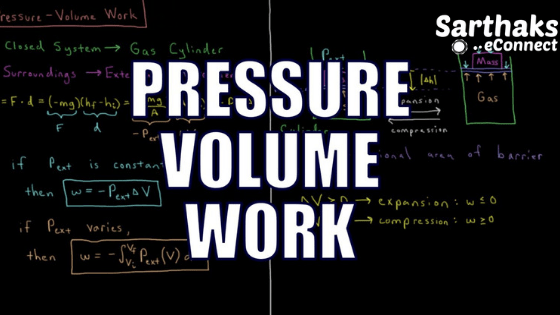• Work of irreversible expansion against constant pressure p under isothermal conditions
q = -Wpv = pext– ΔV
•  Work of reversible expansion under isothermal conditions.But,

PV = RT

Wo=$$\large \int_{v_1}^{v_2 }\frac{RT}{V}dV$$

W=RT$$\large \int_{v_1}^{v_2 }\frac{dV}{V}$$

W=RT$$[loge V]_{V_1}^{V_2}$$

W=RT[$$log_eV_2-log_eV_1$$]

W=2.303RT $${\log_{10}}\frac{V_2}{V_1}$$

Work of reversible expansion under adiabatic conditions.

but,$$\gamma=\frac{C_p}{C_v}$$

$$C_p=\frac{f}{2}R+R$$

$$C_v=\frac{f}{2}R$$

$$\bigtriangleup W=\frac{nR(T_i-T_f)}{\gamma-1}$$

•  Work of irreversible expansion under adiabatic conditions

Wirre = -pext $$\times R[\frac{ p_1T_2-p_2T_1}{p_1p_2}]$$

•  When an ideal gas expands in vacuum then  pext = 0
•  Work done is maximum in reversible conditions
Units: CGS system – erg.
SI system – joule.
•  [Work and heat both appear only at the boundary of the system during a change in state.]

#### Heat Capacity of a System:

• Heat Capacity (c) of a system is the amount of heat required to raise the temperature of a system by 1°C.
• Molar Heat Capacity: It is the heat capacity 1 mole of the substance of the system.
• Specific Heat Capacity: It is the heat capacity of 1 g of the substance of the system
q = mc Δ T,
Where m = mass of substance c = specific heat or heat capacity
• Molar heat capacity, at constant pressure,
Cp = cp $$\times {M}$$
• Molar heat capacity, at constant volume,
Cv = cv $$\times {M}$$
(cp & cv are specific heats at constant pressure & constant volume, M is molecular weight of gas)
cp – cv = R      ( R = Molar gas constant).
C– Cv = $$\frac{R}{M}$$
• The molar heat capacity at constant volume,
Cv = $$\frac{3}{2}R$$
• The molar heat capacity at constant pressure,
• Cp =$$\frac{3}{2}$$R+R = $$\frac{5}{2}$$R
Poisson’s ratio :                      $$\gamma=\frac{C_p}{C_v}=\frac{5}{3}$$
• γ = 1.66 for monoatomic gas,                                                     1.66
•        1.40 for a diatomic gas
•        1.33 for triatomic gas

## Enthalpy (H) in Thermodynamic:•  It is the sum of internal energy and pV-energy of the system. It is a state function and extensive property. Mathematically,
H = U + PV
Like U, the absolute value of H also cannot be known, ΔH is determined experimentally.
ΔH = H2 – H1                     or,
ΣHP = ΣHR
• For exothermic reaction (the reaction in which it evolves heat),     ΔH = -ve whereas
• For endothermic reaction (the reaction in which it absorbs heat),  ΔH = +ve.

### Relationship between ΔH and ΔU:

ΔH = ΔU + Δp ΔV
or,
ΔH = ΔU + Δn(g)RT
Here, Δn(g) = change in the number of gas moles.

#### Enthalpy or Heat of Reaction (ΔrH):

It is the change in enthalpy that accompanies a chemical reaction represented by a balanced chemical equation.
ΔrH = ΣH(p)– ΣH(R)
Enthalpy of reaction expressed at the standard state conditions is the standard enthalpy of reaction (ΔH).

#### Factors affecting enthalpy of reaction:

• Physical state of reactants and products.and
• Allotropic forms of elements involved.
• Chemical composition of reactants & products
• Amount of reactants & Temperature

## Various Forms of Enthalpy of Reaction:1. Enthalpy of Formation (ΔHf): It is heat change when we take one mole of compounds from Its constituent elements. Enthalpy of formation at standard state is known as standard enthalpy of formation ΔfH° and is taken as zero by convention. It also gives stability.
2.  Enthalpy of Combustion (ΔcH): It is the Enthalpy change taking place when one mole of a compound undergoes complete combustion In the presence of oxygen (ΔHc). ΔHc is always negative because process of combustion is exothermic
3.  Enthalpy of Solution (ΔsolH): It is the Enthalpy change when one mole of a substance is dissolved in large excess of solvent so that on further dilution no appreciable heat change occurs.
ΔsolHlattice H + Δhyd H
4. Enthalpy of Hydration (Δhyd H): It is the enthalpy change when one mole of anhydrous substances undergo complete combustion. It is an exothermic process.
5. Enthalpy of Fusion (Δfus H): It is the enthalpy change that accompanies melting of one mole of solid substance.
6. Enthalpy of Vaporisation (Δvap H): It is the enthalpy change that accompanies the conversion of one mole of liquid substance completely into vapors.

### Enthalpy of Neutralisation (Δn H–):

[It is the enthalpy change that takes place when 1 g-equivalent of an acid (or base) is neutralized by 1 g-equivalent of a base (or acid) in dilute solution. Enthalpy of neutralization of strong acid and strong base is always constant, i.e., 57.1 kJ.]
1. [Enthalpy of neutralization of strong acid and weak base or weak acid and strong base is not constant and numerically less than 57.1 kJ due to the fact that here the heat is used up in ionization of weak acid or weak base.It is enthalpy of ionization of weak acid / or base.]
2. Enthalpy of Transition (ΔtH): It is the enthalpy change when one mole of the substance undergoes a transition from one allotropic form to another.
3. Enthalpy of Atomisation (Δa H): It is the enthalpy change occurring when one mole of the molecule breaks into its atoms.
4.  Enthalpy of Dilution It is the enthalpy change when one mole of a substance is diluted from one concentration to another.
5. Enthalpy of Sublimation (Δsub H): It is the enthalpy change when one mole of a solid substance sublimes.
6. Lattice Enthalpy: It is the enthalpy change when one mole of ionic compound dissociates into it’s in gaseous states.
7. Bond Enthalpy(Δbond H): Required to break a bond & energy is released when bond is formed

## Bond Dissociation Enthalpy:

• The enthalpy change is the change in enthalpy when we take one mole of covalent bonds of a gaseous covalent compound which break to form product in the gas phase

### Mean bond Enthalpy:

• The average value of dissociation energies of polyatomic molecules. Some factor affects the bond enthalpy.
• Size of atoms
• Electronegativity
• Bond length
• Number of bonding electrons

## Laws of Thermochemistry in Thermodynamics:• Spontaneous Process: The physical or chemical process that proceeds by its own in a particular direction under a set of conditions without outside help is a spontaneous process. It cannot be reversed.
All-natural processes are spontaneous process.
Spontaneous process where no initiation is needed:
• Sugar dissolves in water
• Evaporation of water
• Nitric oxide (NO) reacts with oxygen.
Spontaneous process where some initiation is required:
• Coal keeps on burning once initiated.
• Heating of CaCO3 to give calcium oxide and CO is initiated by heat.
CaCO3(s)  →   CaO(s)   +  Co2(g)

Enthalpy Criterion of Spontaneous Process: All the processes which are accompanied by decrease of energy (exothermic reactions, having a negative value of ΔH) occur spontaneously. It fails when some endothermic reactions occur spontaneously.

•  Lavoisier Laplace Law:
The enthalpy change during a reaction is equal in magnitude to the enthalpy change in the reverse process but it is opposite in sign.

### Hess’s Law of Constant Heat Summation:The standard enthalpy of a reaction. Which takes place in several steps, is the sum of the standard enthalpies of the intermediate reactions into which the overall reactions may be divided at the same temperature.
According to Hess’s law

ΔH = ΔH1 + ΔH2 + ΔH3

#### Applications of Hess’s law are:

• In the determination of beat of formation.
•   heat of transition. &  hydration.
• To calculate bond energies.
•  Trouton’s Rule:
According to this law, “The ratio of enthalpy of vaporization and normal boiling point of a liquid is approximately equal to 88 J per mol per Kelvin. i.e.,
ΔHvap/T = 88 J /mol /K
• Dulong and Petit Law
This law states “The product of specific heat and molar mass of any metallic element is equal to 6.4 cal/mol/°C.
specific heat * molar mass = 6.4 cal/mol/°C.
• kirchhoff’s Equation
ΔCp= ΔH2 – ΔH1/ T2 – T1 &
ΔCv = ΔE2 – ΔE1/T2 – T1
• Clausius – Clapeyron Equation:
2.303 log p2/p1 = ΔHv/R (T2 – T1/T1*T2)
where ΔHv = molar heat of vaporisation.

## Entropy(S):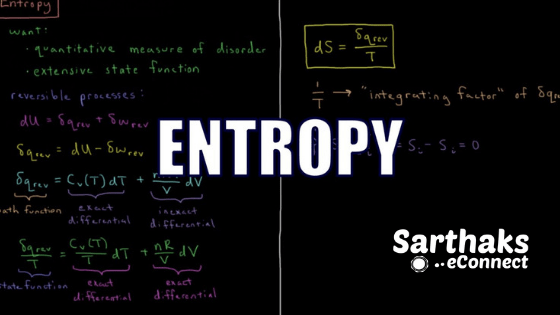• It is a measurement of randomness & disorder of the molecules.
• It is state function & extensive property.
•  Unit: jK-1mol-1
• The change in entropy during a process is :
ΔrS° = ∈S° (product) – ∈S°(reactants) = qrev/T = ΔH/T
Where qrev = heat absorbed by the system in reversible manner
T = Temperature.
ΔS > 0, Increase in randomness, heat is absorbed.
ΔS < 0, Decrease in randomness, heat is evolved.
The entropy of even elementary substances is not 0.
• Entropy change of an ideal gas is:
•  ΔS =nCv ln$$\frac{T_2}{T_1}+ nR ln\frac{V_2}{V_1}$$

Entropy change during phase Transition in Thermodynamics:

Phase transition is the change of matter from one state to another state• The entropy changes at the time of phase transition:
ΔSmelting = ΔHfusion/T
Tm = melting point of substance.
ΔSvapourisation = ΔHvaporisation/Tb
Tb = boiling point of substance.
ΔSsublimation = ΔHsublimation/Tsub
Tsub= sublimation temperature. and
Entropy Criterion of Spontaneous Process:
• A process is spontaneous if and only if the entropy of the universe increases.
For a process to be Spontaneous; (ΔSuniverse > 0 or ΔSsyst + ΔSsurr > 0)
At equilibrium state, ΔS = 0,
Limitations of ΔS criterion and need for another term:
We cannot find an entropy change of surroundings during chemical changes. So we need another parameter for spontaneity Gibbs’ energy of a system (G).

## Second Law of Thermodynamics: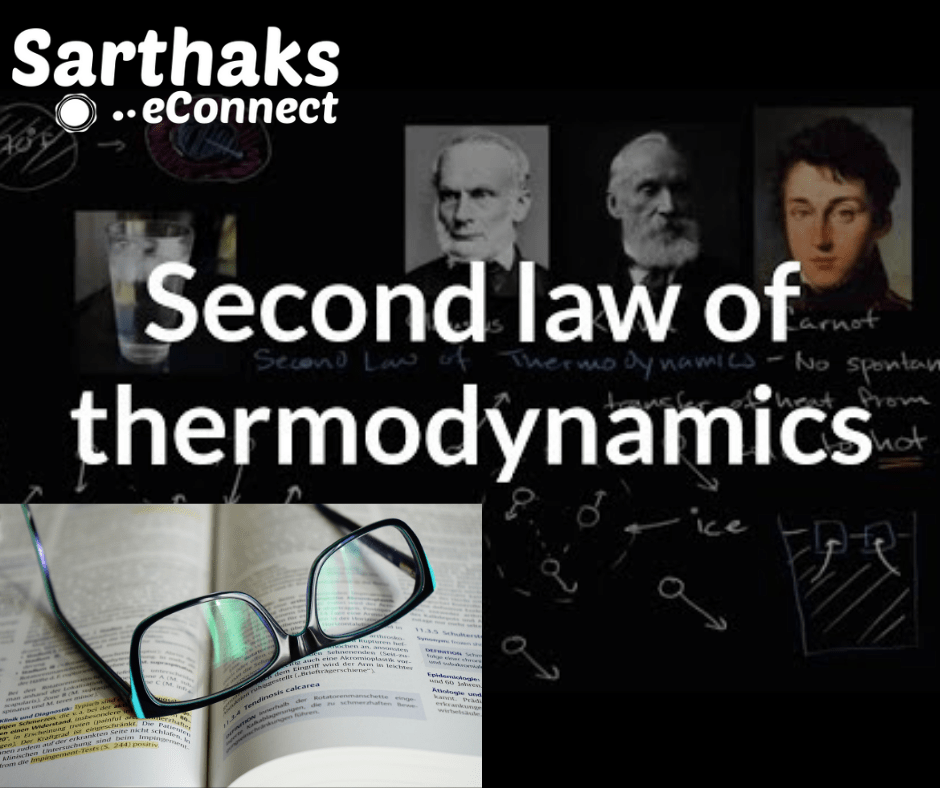The entropy of the universe is always increasing in the course of every spontaneous and natural change.                     Or
All spontaneous processes or natural change are thermodynamically irreversible without the help of an extreme work. i.e., heat cannot flow itself from a colder to hotter body

### Joule-Thomson Effect:

The phenomenon of cooling of a gas when we make it to expand adiabatically from a region of high pressure to a region of extreme low pressure is  Joule-Thomson effect. This effect is zero when an ideal gas expands in vacuum.

• [When an ideal gas undergoes expansion under an adiabatic condition in a vacuum, no change takes place in its internal energy, i.e.,
(∂E / ∂V)T = 0 where   (∂E / ∂V)T is called the Internal pressure.]

#### Joule-Thomson Coefficient:

The number of degrees of temperature change produced per atmospheric drop in pressure at constant enthalpy when a gas to expand through a porous plug is  Joule-Thomson coefficient.They give it as;
μ = dT/dp where,
μ = Joule-Thomson coefficient dT = change in temperature
dp = change in pressure.

### Inversion Temperature:

• The temperature at which a gas becomes cooler on expansions is inversion temperature.
Ti = $$\frac{2a}{Rb}$$ where,
a & b = van der wall constant.
At inversion temperature Ti , the joule –Thomson coefficient μ = 0. The gas neither heated nor cooled.

## Third Law of Thermodynamics:Given by Nernst in 1906.According to this Law “The entropy of a perfectly crystalline substance at zero K or absolute zero is  zero”.
Where,
Cp = heat capacities
T = temperature between 0 K & T K.
This law is only applicable for perfect crystalline substances. If there is imperfection at 0 K, The entropy will larger than zero.

### Carnot Cycle:1. It is an imaginary cycle which demonstrates the maximum conversion of heat into work and it involves four processes.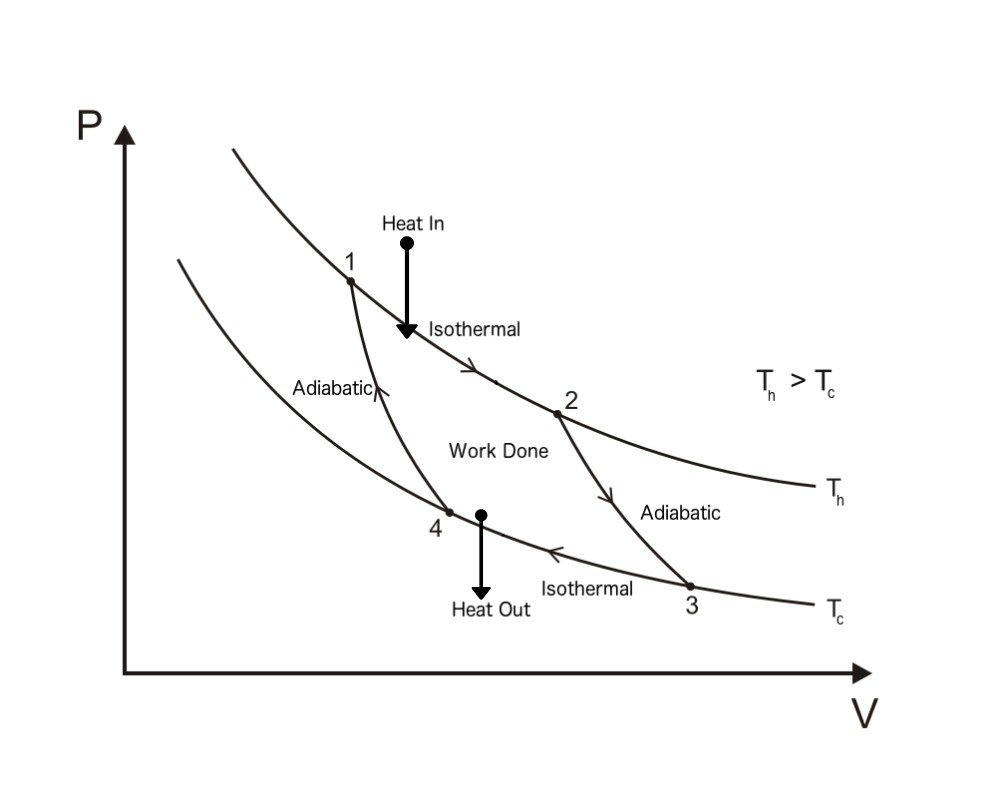(i) Isothermal reversible expansion;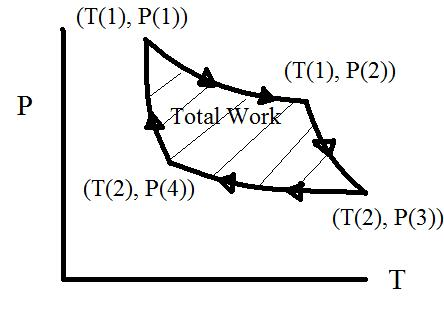The efficiency of a heat engine in a Carnot cycle,
η =$$\frac{{T_2-T_1}}{T_2}$$
=$$\frac{{q_2-q_1}}{q_2}$$=$$\frac{w}{q_2}$$

## Gibbs Energy or Gibbs free energy:

It is the energy available for a system at some conditions and by which useful work can be done and It is a state function and extensive property.

Mathematically,
G = H – TS
Gibbs Helmholtz equation. give Change in Gibbs energy during the process:
(ΔG = G2 – G1 = ΔH – TΔS)
Where, ΔG = Gibbs free energy H = enthalpy of system TΔS = random energy
ΔGsystem = – TΔStotal

### The Gibbs energy criterion of spontaneity

• ΔG > 0, process is non-spontaneous.
• ΔG < 0 process is spontaneous. and
• ΔG = 0,process is in equilibrium state.
• Effect of Temperature on Spontaneity:
• Now an exothermic reaction which is non-spontaneous at high temperature may become spontaneous at low temperature.
• Similarly, endothermic reactions which are non-spontaneous at low temperature and may become spontaneous at high temperature.

## Standard Free Energy Change (ΔG°)

So it is the change in free energy which takes places when the reactants are convert into products at the standard states, i.e., (1 atm and 298 K)

ΔG° = ΔH° – TΔS°

ΔG°reaction = npΔG°products  –  nrΔG°reactants

Where,
ΔG°p= Standard Free Energy of formation

Like Standard Free Energy of formation of all element is 0.

### Gibbs Energy Change and Equilibrium, therefore:

Criterion for equilibrium,

A + B ⇄ C + D
ΔG= 0 Now,
ΔG = ΔG°+ RT ln Q
0 = ΔG°+ RT ln k
ΔG° = – RT ln k
ΔG° = -2.303 RT log K
We know that,
ΔG° = ΔH°– TΔS° = – RT ln K
Relation between ΔG° and EMF of the Cell:
ΔG° = -nFE°Cell
Where,
n = number of electrons lost or gained.
Cell= standard electrode potential.

## Important Question & Answers in Thermodynamics:

1.  For the process to occur under adiabatic conditions, the correct condition is:
(i) ∆T = 0   (ii) ∆p = 0   (iii) q = 0   (iv) w = 0

Hence the correct option is (iii)

Explanation: A system is said to be an adiabatic condition if there is no exchange of heat between the system and its surroundings. Hence q = 0.

2. Two litres of an ideal gas at a pressure of 10 atm expands isothermally at 25 °C into a vacuum until its total volume is 10 litres. How much heat is absorbed and how much work is done in the expansion?

ANS:   We have q = – w  =  pex (10 – 2) =  0(8) = 0
No work is done; no heat is absorbed.

3. we assume If water vapour is to be a perfect gas, the molar enthalpy change for vapourization of 1 mole of water at 1bar and 100°C is 41kJ mol–1. Calculate the internal energy change, when 1 mol of water is vapourised at 1 bar pressure and 100°C.

ANS:

(i)   The change   H2O (l )   →   H20 (g)

∆U = ∆H – ∆ng RT, substituting the values, we get

∆U = 41.00 KJmol-1 * 8.3 Jmol-1K-1  * 373 K

= 41.00 KJmol-1 –  3.096 KJmol-1

=37.904 KJmol-1

4. We subjected a system containing an ideal gas to several changes, as shown in the p-V diagram. In the following cyclic process, A to F the type of change taking place from B to C. given that.  The temperature at A, B & F = T1  Temperature at C, D and E = T2

a. Isochoric and isothermal (pressure falls)
b. Adiabatic compression, temperature increases to T1
c. Adiabatic expansion, temperature fall to T2
d. Isochoric and isothermal (pressure increases